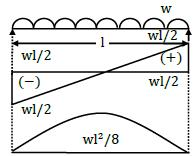Courses

# Test: SFD & BMD Level - 1

## 20 Questions MCQ Test Strength of Materials (SOM) | Test: SFD & BMD Level - 1

Description
This mock test of Test: SFD & BMD Level - 1 for Mechanical Engineering helps you for every Mechanical Engineering entrance exam. This contains 20 Multiple Choice Questions for Mechanical Engineering Test: SFD & BMD Level - 1 (mcq) to study with solutions a complete question bank. The solved questions answers in this Test: SFD & BMD Level - 1 quiz give you a good mix of easy questions and tough questions. Mechanical Engineering students definitely take this Test: SFD & BMD Level - 1 exercise for a better result in the exam. You can find other Test: SFD & BMD Level - 1 extra questions, long questions & short questions for Mechanical Engineering on EduRev as well by searching above.
QUESTION: 1

Solution:
QUESTION: 2

Solution:
QUESTION: 3

### Between point loads the shear varies

Solution: Shear force is constant between two point loads.

QUESTION: 4

The shear force diagram for a cantilever subjected to uniformly distributed load over its entire span is

Solution: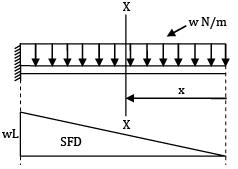SFX−X = wx

QUESTION: 5

A beam is said to be in general stable and statically determinate for general loading when number of reaction components is

Solution:
QUESTION: 6

The reaction at a hinged support of a beam is

Solution:
QUESTION: 7

For overhanging beams, the point of contra flexure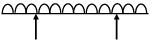Solution: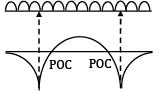→ Point of contraflexure is a point where bending moment changes its sign.

→ As from the BMD it is clear that in between the supports the BM is changing the sign.

QUESTION: 8

At a point of contra flexure

Solution: It is the point where the BM is zero after changing its sign from positive to negative or vice versa.

QUESTION: 9

Maximum bending moment occurs in simply supported beam, where the [

Solution:
QUESTION: 10

Solution: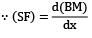QUESTION: 11

For a beam subjected to a constant bending moment throughout its length

Solution:
QUESTION: 12

The difference in ordinate of the shear curve between any two sections is equal to the area under

Solution:
QUESTION: 13

A lever is supported on two hinges at A and C. It carries a force of 3 kN as shown in the above figure. The bending moment at B will be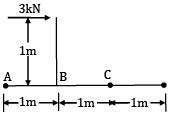Solution: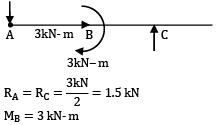QUESTION: 14

The shear force at the free end of an overhanging beam (with udl) is equal to

Solution: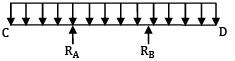Shear force will increase linearly from end D to support B starting from zero.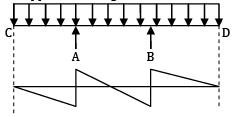QUESTION: 15

A wooden log of length 5mm is floating in water. First it’s self-weight is 1kN/m. The maximum B.M at the center is

Solution: Due to self-weight SF and BM will not occur when the member is freely floating.

QUESTION: 16

Bending moment in a simply supported beam is maximum at

Solution:
QUESTION: 17

Bending moment in a cantilever beam shown below will be zero at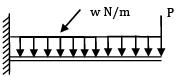Solution:
QUESTION: 18

When a SSB is subjected to a concentrated moment at a distance of L/4 from the right end, the maximum bending moment occurs at

Solution:
QUESTION: 19

Shear force at any section is the algebraic sum of the following force on the either side

Solution: Shear force at any point or at any section is the algebraic sum of transverse shear forces either to the left or to the right of a section.

QUESTION: 20

For a simply supported beam of length ‘l’ subjected to downward load of uniform intensity w,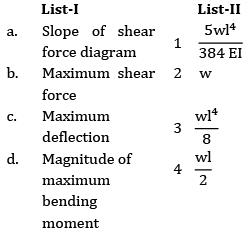Codes: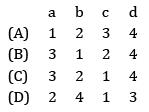Solution: